# Economics Study Set 17

## Quiz 26 : Demand-Side Equilibrium: Unemployment or InflationStudy FlashcardsLooking for Economics Homework Help?

## Quiz 26 :Demand-Side Equilibrium: Unemployment or Inflation

Question TypeUse both numerical and graphical methods to find the multiplier effect of the following shift in the consumption function in an economy in which investment is always $220, government purchases are always$100, and net exports are always -$40. (Hint: What is the marginal propensity to consume )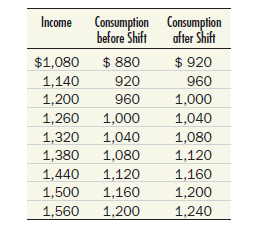Free Essay Answer: Answer: Graphical presentation of equilibrium level of GDP In Figure (1), GDP is measured on horizontal axis and consumption expenditure is measured on vertical axis.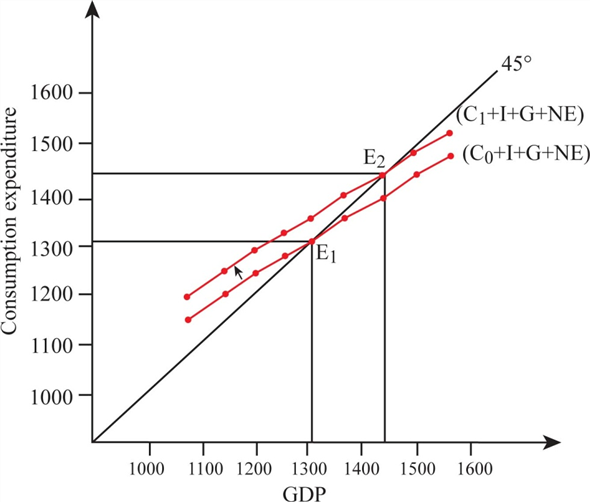Figure - 1 The increase in consumption shifts the consumption expenditure curve upward from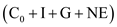to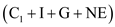. As a result, the new equilibrium level of GDP is obtained at point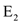where GDP and consumption expenditure intersect the 45-degree line. As the consumption increases by$40, the consumption expenditure schedule increases and GDP and consumption expenditure obtain equilibrium when both are at $1,440. Hence, the consumption multiplier effect is$120.

Tags
Choose question tag(More difficult) 9 Consider an economy in which the consumption function takes the following simple algebraic form: C = 300 + 0.75 DI and in which investment ( I ) is always $900 and net exports are always -$100. Government purchases are fixed at $1,300 and axes are fixed at$1,200. Find the equilibrium level of GDP, and then compare your answer to Table 1 and Figure 2. ( Hint: Remember that disposable income is GDP minus taxes: DI = Y - T = Y - 1,200.)
Free
Essay

An equilibrium level of GDP of an economy can be derived using the following method: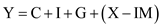……(2)
Substitute the respective values in Equation (2), to obtain the equilibrium level of GDP.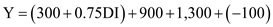Since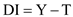, substitute Y and T in the above equation.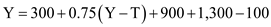Since T is $1,200, the value of T is substituted in the above equation and rewritten as follows: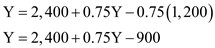To simply the equation, bring 0.75Y to the left-hand side of the equation. Hence, the equation can be written asHence , the equilibrium level of GDP demanded in an economy is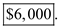Comparison of derived result with Table-1 and Figure-2 from the book. The derived level of GDP is$6,000, which is equal to the total expenditure given in Table-1and Figure-2.
Hence, the comparison of the derived result indicates an equilibrium level of GDP.

Tags
Choose question tagFor about 30 years now, imports have consistently exceeded exports in the U.S. economy. Many people consider this imbalance to be a major problem. Does this chapter give you any hints about why (You may want to discuss this issue with your instructor. You will learn more about it in later chapters.)
Free
Essay

If the imports of an economy consistently exceed the exports of an economy, then the net exports will be negative.
Negative net exports will push down the equilibrium level of GDP
Based on the discussion in the chapter, increase in net exports will have a multiplier effect on GDP. It means a small increase in net exports will cause an increase in GDP at a higher rate. On the other hand, a small fall in net exports will cause the GDP to fall at a higher rate.
Thereby, the multiplier effect of negative net exports will push the equilibrium level of GDP down.

Tags
Choose question tagFrom the following data, construct an expenditure schedule on a piece of graph paper. Then use the income-expenditure (45° line) diagram to determine the equilibrium level of GDP.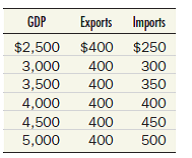Now suppose investment spending rises to $260, and the price level is fixed. By how much will equilibrium GDP increase Derive the answer both numerically and graphically. Essay Answer: Tags Choose question tagIn each of these cases, how much saving is there in equilibrium ( Hint: Income not consumed must be saved.) Is saving equal to investment Essay Answer: Tags Choose question tagFind the equilibrium level of GDP demanded in an economy in which investment is always$300, net exports are always -$50, the government budget is balanced with purchases and taxes both equal to$400, and the consumption function is described by the following algebraic equation: C = 150 + 0.75 DI ( Hint: Do not forget that DI = Y - T. )
Essay
Tags
Choose question tagReferring to Test Yourself Question 1, do the same for an economy in which investment is $250, net exports are zero, government purchases and taxes are both$400, and the consumption function is as follows: C = 250 + 0.5 DI Reference Test Yourself Question 1, Find the equilibrium level of GDP demanded in an economy in which investment is always $300, net exports are always -$50, the government budget is balanced with purchases and taxes both equal to $400, and the consumption function is described by the following algebraic equation: C = 150 + 0.75 DI ( Hint: Do not forget that DI = Y - T. ) Essay Answer: Tags Choose question tagFredonia has the following consumption function: C = 100 + 0.8 DI Firms in Fredonia always invest$700 and net exports are zero, initially. The government budget is balanced with spending and taxes both equal to $500. a. Find the equilibrium level of GDP. b. How much is saved Is saving equal to investment c. Now suppose that an export-promotion drive succeeds in raising net exports to$100. Answer (a) and (b) under these new circumstances.
Essay
Tags
Choose question tagSuppose that investment spending is always $250, government purchases are$100, net exports are always $50, and consumer spending depends on the price level in the following way: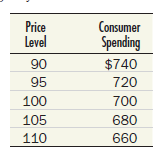On a piece of graph paper, use these data to construct an aggregate demand curve. Why do you think this example supposes that consumption declines as the price level rises Essay Answer: Tags Choose question tagSuppose exports and imports of a country are given by the following:Calculate net exports at each level of GDP. Essay Answer: Tags Choose question tag(More difficult) What would happen to the multiplier analysis if b = 0 If b = 1 Essay Answer: Tags Choose question tag(More difficult) An economy has the following consumption function: C = 200 + 0.8 DI The government budget is balanced, with government purchases and taxes both fixed at$1,000. Net exports are $100. Investment is$600. Find equilibrium GDP. What is the multiplier for this economy If G rises by $100, what happens to Y What happens to Y if both G and T rise by$100 at the same time
Essay
Tags
Choose question tagExplain the basic logic behind the multiplier in words. Why does it require b, the marginal propensity to consume, to be between 0 and 1
Essay
Tags
Choose question tagTry to remember where you last spent a dollar. Explain how this dollar will lead to a multiplier chain of increased income and spending. (Who received the dollar What will he or she do with it )
Essay
Tags
Choose question tagImagine an economy in which consumer expenditure is represented by the following equation: C = 50 + 0.75 DI Imagine also that investors want to spend $500 at every level of income ( I =$500), net exports are zero ( X - IM = 0), government purchases are $300, and taxes are$200. a. What is the equilibrium level of GDP b. If potential GDP is $3,000, is there a recessionary or inflationary gap If so, how much c. What will happen to the equilibrium level of GDP if investors become optimistic about the country's future and raise their investment to$600 d. After investment has increased to $600, is there a recessionary or inflationary gap How much Essay Answer: Tags Choose question tagDoes the economy this year seem to have an inflationary gap or a recessionary gap (If you do not know the answer from reading the newspaper, ask your instructor.) Essay Answer: Tags Choose question tagIf domestic expenditure (the sum of C + I + G in the economy described in Test Yourself Question 1) is as shown in the following table, construct a 45° line diagram and locate the equilibrium level of GDP.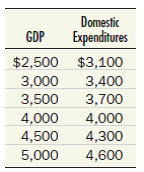Essay Answer: Tags Choose question tagExplain the logic behind the finding that variable imports reduce the numerical value of the multiplier. Essay Answer: Tags Choose question tagLook back at the income-expenditure diagram in Figure 3 and explain why some level of real GDP other than$6,000 (say, $5,000 or$7,000) is not an equilibrium on the demand side of the economy. Do not give a mechanical answer to this question. Explain the economic mechanism involved.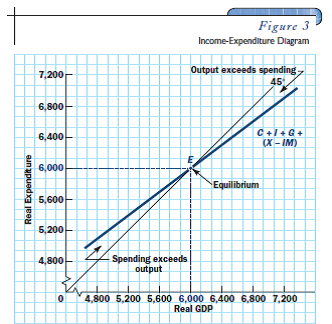EssayFrom the following data, construct an expenditure schedule on a piece of graph paper. Then use the income-expenditure (45° line) diagram to determine the equilibrium level of GDP. Compare your answer with your answer to the previous question.